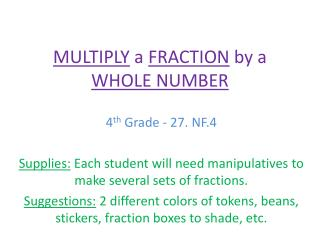DownloadDownload PresentationMULTIPLY a FRACTION by a WHOLE NUMBER

# MULTIPLY a FRACTION by a WHOLE NUMBER

Télécharger la présentation## MULTIPLY a FRACTION by a WHOLE NUMBER

- - - - - - - - - - - - - - - - - - - - - - - - - - - E N D - - - - - - - - - - - - - - - - - - - - - - - - - - -
##### Presentation Transcript

1. MULTIPLY a FRACTION by a WHOLE NUMBER 4th Grade - 27. NF.4 Supplies: Each student will need manipulativesto make several sets of fractions. Suggestions: 2 different colors of tokens, beans, stickers, fraction boxes to shade, etc.

2. Our Problem is: 5 x (1/4) = ___ • How many ¼ do we have? • 5 • Show me 5 separate representations of ¼. • XXXX(1/4) XXXX(1/4) XXXX(1/4) XXXX(1/4) XXXX(1/4) • How do we find out how many ¼ we have altogether? • Repeated Addition • ¼ + ¼ + ¼ + ¼ + ¼ = 5/4 • Now put all your representations of ¼ together. • X XXXX (5/4) • Can we leave the answer as 5/4? • NO

3. How do we rename the improper fraction 5/4? • Change it into a mixed number • How do we make this fraction a mixed number? • Divide • How many groups of 4 are there in 5? • 1 • Are there any ¼ remaining? • Yes, we have one ¼ remaining. • What is our final answer? • 1 ¼ CONGRATULATIONS!!! •  Let’s try another problem!!!  6 X (1/7) = ___